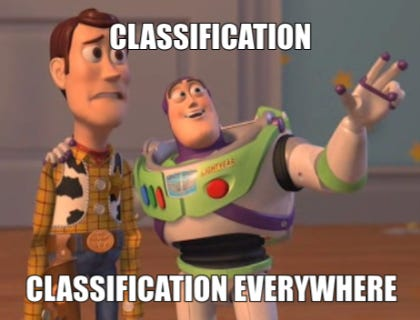# Deep into Learning : 3. Classification

Source: Deep Learning on Medium

What is it? As the name suggest , classify stuffs with the given data.

For example, I have a data of Hours of study students put on preparinf for exams , their CGPA and each data have a label if they have a life or not. 😝(6 pointer here).

So our Imaginery data is,
X₁ = Hours of study
X₂ = CGPA
y = label , is 0 if the students life sucks, 1 😁 if its amazing(like me 😅 ).

Our target is to predict if y is 0/1 for a given student , given his/her CGPA, Study time.

So this is a classification problem, with the given data we not classify if a new student(given their CGPA, study time) fall into category 1 or 0.

So our data may look like this,

Our target in classification problem is to make a line/boundary which can classify the data . Here is our data point lies above the line it, then y=1(the guy/girl should get a life), if its below the line then y=0.

so our line can be w₁x₁ + w₂x₂ + b = 0 , out task is to get optimal w₁, w₂, b so that it classifies the data better.

We will see about how to predict the boundary to classify the data later.

What if our data have more than 2 features say 3(for easy visualization) it can 4,5,6…..1000….10000….

So lets take the data as CGPA, Study Time and Gaming time, and label is same y=0/1.

Now we have 3rd dimension(Gaming time) in our data. So how do we seperate/classify the data.

Plane!!

Our data may look something like this

our plane will be w₁x₁ + w₂x₂ +w₃x₃+ b = 0, so we have to find optimal w₁, w₂, w₃, b so that the plane classifies the data better.

for even higher dimension, w₁x₁ + w₂x₂ +w₃x₃+……+wₙxₙ+ b = 0, so we need to find optimal w₁, w₂, ….. wₙ, b . Now our data will need a n-1 dimensional hyperplane to classify our data(which is beyond our visual imagination , but we can compute it using vectors and matrix).

From next post, let’s start to learn how to find this hyperplane which classifies our data.

Feel free to suggest/critic , this series on Deep Learning is for my personal experience and hope it may help someone.

See you in the next post.

Contact me